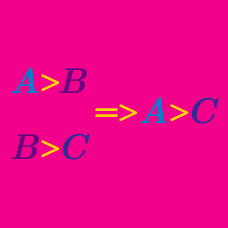Logic

# Logical Reasoning: Level 1 Challenges

John, Aries, and Joseph are brothers with different ages. Who is the youngest if the following statements are true?

I. Aries is the oldest.
II. Joseph is not the oldest.
III. John is not the youngest.

Rony is taller than Joy and John is shorter than Rony.
Which of the following statements is correct?

Think of any number. Multiply it by 6 and add 3. Then divide it by 3 and add 8. Lastly, subtract twice the original number. I know what number you have left. What must it be?O, P, Q, R, S and T are standing on a bench according to their height. P is taller than O but shorter than S. Only S is taller than T. R is shorter than P but taller than Q. Who is the tallest?

Think of any positive number. Multiply it by itself and subtract negative 3 from it. Then add the original number four times. Divide your resultant number by one more than the original number. Lastly, take the resultant number and subtract off the original number. I know what number you have. What must it be?

×

Problem Loading...

Note Loading...

Set Loading...